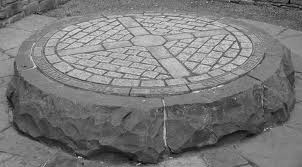Miracles of the Qur'an# SURAS THAT HAVE INITIAL LETTERS AND 19

The Quran’s initial letters have been one of its more curious aspects. Some suras are introduced with initial letters like “Qaf” and “Alif-Lam-Meem:” What the initial letters represent was a mystery until the manifestation of code 19. When code 19 became manifest, it was understood that the initial letters had a significant role with reference to the miracle of 19. You can see the suras and verses with initial letters in the Quran in the table below:

There are 29 suras with initial letters and the total number of verses having initial letters is 30. We mentioned before that 30 was the 19th composite number. The initial letters themselves form independent verses without any other words in 19 of those 29 suras (for example, “Alif, Lam, Meem” is a verse in itself in the sura “The Cow”). In the other 10 suras, there are some words after the initial letters (for example, the first verse of the sura “Qaf” states, “Qaf, the Glorious Quran”).

 The number of suras with initial letters as verse 19

The verses draw attention to the Quran (by using the words “Quran” or “Book”) in 9 out of 10 suras that have initial letters together with words. The total of the sura numbers of these 9 suras is the number “190” (10+11+12+13+14+15+27+38+50= 190), and 190 is equal to 10 x 19.

 The total number of initial lettered suras that refer to the Quran together with initial letters 190 (19×10)

The initial letters can be grouped according to their repetitions. One group consists of initial letters used only once, and the other group consists of initial letters repeated more than once. The initial letters repeated more than once consist of four groups: “Alif, Lam, Meem,” “Ta, Seen, Meem,” “Ha, Meem,” “Alif, Lam, Ra.” The number of verses belonging to the suras having these 4 groups of initial letters is 1900 (19 x 100).

 The total of the verses in suras which have the repeated initial letter groups 1900 (19×100)

There are 67 suras between the second sura of the Quran, which has the first initial letters (2nd sura), and the 68th sura, which has the last initial letters. The number 67 is the 19th prime number. Because 29 of these 67 suras begin with initial letters, there are only 67- 29 = 38 (19×2) suras that are not introduced with initial letters between the suras with initial letters.

 The suras that do not have initial letters, between the suras with initial letters 38 (19×2)

We mentioned that we were not going to deal with the counting of the letter “Alif” in the Quran since there is difficulty in its counting. The difficulty is due to the additional “Alif”s inserted in later versions of the Quran to accommodate non-Arabs, and the need for a comprehensive, comparative and critical study of the oldest available versions. However, we can show that the suras introduced with the initial letter “Alif” are related to the code 19. For instance, the total of the sura numbers of the suras starting with the initial letter “Alif” is an exact multiple of the number 19.
[2+3+7+10+11+12+13+14+15+29+30+31+32 = 209 (19×11)]

 The total of the verse numbers where the letter “Alif” is used as initial letter 209 (19×11)

If we examine the number of verses in the suras that begin with initial letters, we see that the 28th and 38th suras consist of 88 verses each, and the 14th and 68th suras consist of 52 verses each. Thus, we see that there are 27 different numbers in the number of the verses of the suras starting with initial letters. The total number of the verses, excluding repetitions, is 2603 (19×137).

 The total of the verses in the initial lettered suras, excluding repetitions 2603 (19×137)

There are many characteristics that are related to the code 19 in the suras introduced with initial letters. All these examples show that the row of the suras and the number of verses in the Quran are arranged in a given order. There are 14 combinations of initial letters in the Quran and the total number of initial letters in these combinations is 38 (19×2). And if we multiply the serial numbers of these combinations and the number of the initial letters in each combination and then add all these results, it makes up 247 (19×13). Look at the following table:

 Row number Initial letters Number of letters in the group The multiplication of the row number and the number of letters 1 Alif-Lam-Meem 3 1×3=3 2 Alif-Lam-Meem-Sad 4 2×4=8 3 Alif-Lam-Ra 3 3×3=9 4 Alif-Lam-Meem-Ra 4 4×4=16 5 Kaf-He-Ya-Ayn-Sad 5 5×5=25 6 Ta-He 2 6×2=12 7 Ta-Seen-Meem 3 7×3=21 8 Ta-Seen 2 8×2=16 9 Ya-Seen 2 9×2=18 10 Sad 1 10×1=10 11 Ha-Meem 2 11×2=22 12 Ha-Meem-Ayn-Seen-Qaf 5 12×5=60 13 Qaf 1 13×1=13 14 Noon 1 14×1=14 Total 38 (19×2) 247(19×13)

An Iranian scholar named Asad also discovered the interesting fact that there are a total of 114 (19×6) verses in which all the 14 letters that are used in the initial lettered suras are present, like the numbers of the suras of the Quran. In these mentioned 114 verses, the most repeated word of the Quran “God” is used 152 (19×8) times exactly.

 The number of verses in which all the 14 letters that are used in the initial lettered suras, are present. 114 (19×6)

1.2.3.